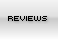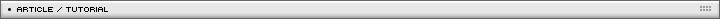Game creation tutorial Page 1/1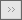[ February 26, 2007 ] Emanuele Feronato This is the third part of a long step-by-step article that will guide you through the entire process of creating a Flash game including input management, basic physics, collision detection and a lot more!

Here we go with the 3rd step.

Remember to read 1st and 2nd part if you are new to this tutorial, and let's go.

We left our hero colleting coins, so next step will be...

The score

Scoring system consist in a variable set to 0 (zero) when the game starts and some events that may increase/decrease the score.

In this case, you get 1 point when you collect a coin, and lose 2 points when you crash into a wall.

Hall I have to do is initializing a variable in the root with a single action

`score = 0;`

And then in the hero movieclip

```  onClipEvent (load) {
yspeed = 0;
xspeed = 0;
wind = 0.00;
power = 0.65;
gravity = 0.1;
upconstant = 0.75;
friction = 0.99;
}
onClipEvent (enterFrame) {
if (Key.isDown(Key.LEFT)) {
xspeed = xspeed-power;
}
if (Key.isDown(Key.RIGHT)) {
xspeed = xspeed+power;
}
if (Key.isDown(Key.UP)) {
yspeed = yspeed-power*upconstant;
}
if (Key.isDown(Key.DOWN)) {
yspeed = yspeed+power*upconstant;
}
xspeed = (xspeed+wind)*friction;
yspeed = yspeed+gravity;
_y = _y+yspeed;
_x = _x+xspeed;
_rotation = _rotation+xspeed;
if (_root.wall.hitTest(_x, _y, true)) {
xspeed = 0;
yspeed = 0;
_x = 120;
_y = 120;
_root.score -=2;
}
if (_root.coin.hitTest(this.hero_hit)) {
_root.coin._x = Math.random()*400+50;
_root.score ++;
}
}
```

Look at lines 33 and 37... as said, you get one point when you collect a coin and lose 2 points when you hit the wall.
A dynamic text in the main scene displays the score.

Sooooo easy... now let me introduce you the main event for this tutorial... the environment...

We can have different kinds of environment... let's start with the easiest.

The killing fixed environment

The killing fixed environment is a deadly part of the stage that does not move, such as a spike.

So I created a new movieclip called environment and instanced as environment and placed in the stage.
You choose how to detect the collision, with the center of the hero or with the hero_hit shape (time to read part 2 if you do not understand what I am talking about).

Just remember you game does not have to be too easy or too hard.

``` onClipEvent (load) {
yspeed = 0;
xspeed = 0;
wind = 0.00;
power = 0.65;
gravity = 0.1;
upconstant = 0.75;
friction = 0.99;
}
onClipEvent (enterFrame) {
if (Key.isDown(Key.LEFT)) {
xspeed = xspeed-power;
}
if (Key.isDown(Key.RIGHT)) {
xspeed = xspeed+power;
}
if (Key.isDown(Key.UP)) {
yspeed = yspeed-power*upconstant;
}
if (Key.isDown(Key.DOWN)) {
yspeed = yspeed+power*upconstant;
}
xspeed = (xspeed+wind)*friction;
yspeed = yspeed+gravity;
_y = _y+yspeed;
_x = _x+xspeed;
_rotation = _rotation+xspeed;
if (_root.wall.hitTest(_x, _y, true)) {
xspeed = 0;
yspeed = 0;
_x = 50;
_y = 50;
}
if (_root.environment.hitTest(_x, _y, true)) {
xspeed = 0;
yspeed = 0;
_x = 50;
_y = 50;
}
}
```

Lines 34-39 do the routine that checks collision between the hero and the environment

This is useful if you want to place objects on the stage that may kill the hero.

Sometimes, the environment should move.

The killing tweened environment

I am calling "tweened environment" an object that always moves along a path... such as a sliding wall or something similar.

The actionscript, obviously, is the same as above, the only change is to the environment object, that now has a tweening motion. You'll see it better when you will download the sources, at the end of this tutorial.

The only thing I want you to know is: for a better result, the number of pixels the environment moves at every frame should be an integer. I mean that if you want to move your environment by 150 pixels, be sure that dividing the pixels for the numbers of frames you have an integer number.
In my case, I move the environment by 150 pixels in 75 frames... 150/75 = 2 (integer) pixels/frame.
It's not a mandatory rule, and in most cases you will experience it is impossible to achieve such integer numbers, while you can do it, well, do it.

Sometimes we want the hero to interact with environment, so I will explain howo to get...

The triggered environment

Let's suppose to have a wall that moves very fast... making our life so hard... we should let the player decide to cross the wall, risking his life, or turn off the "engine" that moves the wall, for example pushing a button or pulling a lever.

In this new movie I created a new object named as trigger and instanced as trig, with the shape of a lever.

Then I added some actionscript to the environment, that is no longer tweened.

``` onClipEvent (load) {
dir = 4;
moving = 1;
}
onClipEvent (enterFrame) {
if (moving) {
_y += dir;
if (_y > 300) {
dir = -4;
}
if (_y < 50) {
dir = 4;
}
}
}
```

This is a very simple movement routine... I will explain more complex routines later in the tutorial, but at the moment I only want a wall to cross the stage up and down, and the hero to get the trigger to stop it.

The script is very simple, just take a look at the moving variable. You can see that the environment will move by 4 pixels (integer...) upwards or downwards as long as the moving variable is set to 1.

How can we stop it?

With lines 40-43 of the hero's actionscript

``` onClipEvent (load) {
yspeed = 0;
xspeed = 0;
wind = 0.00;
power = 0.65;
gravity = 0.1;
upconstant = 0.75;
friction = 0.99;
}
onClipEvent (enterFrame) {
if (Key.isDown(Key.LEFT)) {
xspeed = xspeed-power;
}
if (Key.isDown(Key.RIGHT)) {
xspeed = xspeed+power;
}
if (Key.isDown(Key.UP)) {
yspeed = yspeed-power*upconstant;
}
if (Key.isDown(Key.DOWN)) {
yspeed = yspeed+power*upconstant;
}
xspeed = (xspeed+wind)*friction;
yspeed = yspeed+gravity;
_y = _y+yspeed;
_x = _x+xspeed;
_rotation = _rotation+xspeed;
if (_root.wall.hitTest(_x, _y, true)) {
xspeed = 0;
yspeed = 0;
_x = 450;
_y = 50;
}
if (_root.environment.hitTest(_x, _y, true)) {
xspeed = 0;
yspeed = 0;
_x = 450;
_y = 50;
}
if (_root.trig.hitTest(_x, _y, true)) {
_root.trig.gotoAndStop(2);
_root.environment.moving = 0;
}
}
```

If the hero hits the trigger (as explained before, you will decide the type of collision checking later when you will test the entire game), the trigger frame is moved to 2 displaying a pulled lever and the moving variable is set to 0.
In this case, the actionscript of the environment won't enter in the if condition and the environment will stop.

Possibile applications of this routine are almost infinite: imagine buttons that stops dangerous object moving, levers that reveals hidden zones with treasures, and so on.

We will discover all those options when the game will be in progress... remember that at the moment I am just showing you the basics.

Talking about basics, let's see a type of environment that as far as I know is not included in ball:revamped (of course we always have to try to improve existing concepts).

The undeadly environment

I want an environment that does not kill the hero, but that changes some behaviours... imagine a stage that is partially flooded.
You have water in the stage, and your hero underwater movements will be more difficult.

```onClipEvent (load) {
yspeed = 0;
xspeed = 0;
wind = 0.00;
power = 0.65;
gravity = 0.1;
upconstant = 0.75;
friction = 0.99;
sunken = 0;
}
onClipEvent (enterFrame) {
if (Key.isDown(Key.LEFT)) {
xspeed = xspeed-power;
}
if (Key.isDown(Key.RIGHT)) {
xspeed = xspeed+power;
}
if (Key.isDown(Key.UP)) {
yspeed = yspeed-power*upconstant;
}
if (Key.isDown(Key.DOWN)) {
yspeed = yspeed+power*upconstant;
}
xspeed = (xspeed+wind)*friction;
yspeed = yspeed+gravity;
if(sunken == 0){
_y = _y+yspeed;
_x = _x+xspeed;
}
else{
_y = _y+(yspeed/5);
_x = _x+(xspeed/3);
}
_rotation = _rotation+xspeed;
if (_root.wall.hitTest(_x, _y, true)) {
xspeed = 0;
yspeed = 0;
_x = 50;
_y = 50;
}
if (_root.environment.hitTest(_x, _y, true)) {
sunken = 1;
}
else{
sunken = 0;
}
}
```

Line 9 has a new variable that determines if the hero is underwater or not.
Then, when it's time to update hero's position according to gravity, speed, and so on...
Lines 26-33 checks if the hero is underwater or not. If it's underwater, movements are reduced by 5 vertically and by 3 horizontally.

Note: I said movements are reduced, not the speed! This means that when you will pop out of the water, you have to pay attention to your speed. I want the player to move carefully when underwater, not simply have slower (easier) movements.

Now let's imagine to have some special object on the stage. If the hero collects such object will raise the score, but the entire environment will get harder.

The playing environment

A little game covering some of the thems we discussed until now.
You have to score as much as you can. As seen before, you get 1 point when you collect a coin, you lose 2 points when you hit the walls and 1 point when you hit the environment.
Easy? Not at all... I forgot to tell you the environment moves, and the higher your score, the faster the movement.

The actionscript on the first frame is

`score = 0;`

You may ask: can't you put this line in the hero onCLipEvent(load)?

Yes, I could, but you will see why I put it there when we will learn how to move around levels.

Anyway, in the environment object we have the actionscript:

```onClipEvent (enterFrame) {
_rotation=_rotation+0.25+_root.score*0.25;
}
```

Do you see? the environment rotation speed increases as long as your score increases. So bad!

Finally, the hero actionscript

``` onClipEvent (load) {
yspeed = 0;
xspeed = 0;
wind = 0.00;
power = 0.65;
gravity = 0.1;
upconstant = 0.75;
friction = 0.99;
_root.coin._x = Math.random()*400+50;
_root.coin._y = Math.random()*250+50;
}
onClipEvent (enterFrame) {
if (Key.isDown(Key.LEFT)) {
xspeed = xspeed-power;
}
if (Key.isDown(Key.RIGHT)) {
xspeed = xspeed+power;
}
if (Key.isDown(Key.UP)) {
yspeed = yspeed-power*upconstant;
}
if (Key.isDown(Key.DOWN)) {
yspeed = yspeed+power*upconstant;
}
xspeed = (xspeed+wind)*friction;
yspeed = yspeed+gravity;
_y = _y+yspeed;
_x = _x+xspeed;
_rotation = _rotation+xspeed;
if (_root.wall.hitTest(_x, _y, true)) {
xspeed = 0;
yspeed = 0;
_x = 50;
_y = 50;
_root.score -= 2;
if (_root.score < 0) {
_root.score = 0;
}
}
if (_root.environment.hitTest(_x, _y, true)) {
xspeed = 0;
yspeed = 0;
_x = 50;
_y = 50;
_root.score -= 1;
if (_root.score < 0) {
_root.score = 0;
}
}
if (_root.coin.hitTest(this.hero_hit)) {
_root.coin._x = Math.random()*400+50;
_root.coin._y = Math.random()*250+50;
_root.score++;
}
}
```

Nothing new, just a check to the score to prevent it to be less than zero.

If you want, drop a comment with the highest score you achieved. I bet you won't easily score more than 15.

Download the examples source code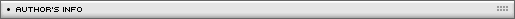Name: Emanuele Feronato Location: Venice, Italy Age: 35 Flash experience: 2 years Job: Web developer and programming teacher Website: http://www.emanueleferonato.com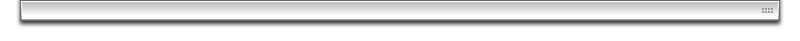| Homepage | News | Games | Articles | Multiplayer Central | Reviews | Spotlight | Forums | Info | Links | Contact us | Advertise | Credits || www.smartfoxserver.com | www.gotoandplay.biz | www.openspace-engine.com | gotoAndPlay() v 3.0.0 -- (c)2003-2008 gotoAndPlay() Team -- P.IVA 03121770048